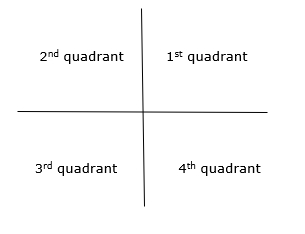# C program to find in which quadrant the coordinates lie.

CServer Side ProgrammingProgramming

## Problem

Write a program to find the quadrant in which the given coordinates lie.

User has to enter a coordinate at runtime and we need to find the quadrant in which these coordinates lie.

## Solution

• If both numbers are positive then, it displays the first quadrant.
Example: Input =2, 3
Output = 1st quadrant
• If the first number is negative and the second number is positive then, it displays the second quadrant.
Example: Input = -4, 3
Output= 2nd quadrant
• If the first number is negative and the second number is also negative then, it displays the third quadrant.
Example: Input = -5,-7
Output= 3rd quadrant
• If the first number is positive and the second number is negative then, it displays the fourth quadrant.
Example: Input = 3,-5
Output = 4th quadrant## Example

Following is the C program to find the quadrant in which the given coordinates lie −

Live Demo

#include <stdio.h>
int main(){
int a,b;
printf("enter two coordinates:");
scanf("%d %d",&a,&b);
if(a > 0 && b > 0)
else if(a < 0 && b > 0)
else if(a < 0 && b < 0)
else if(a > 0 && b < 0)
else
printf("Origin");
return 0;
}

## Output

When the above program is executed, it produces the following output −

Run 1:
enter two coordinates:-4 6
3rd Quadrant